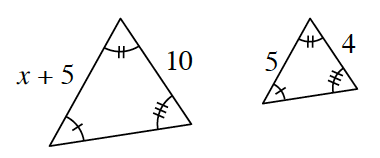### Home > CCG > Chapter 6 > Lesson 6.1.4 > Problem6-37

6-37.Examine the triangles at right. Solve for $x$. Redraw and label the diagrams as needed.

Decide if the triangles are similar. Review the Math Notes boxes in Lessons 3.2.1 and 3.2.5 for help.

Decide which sides of the triangles correspond.

Write a proportion and solve for $x$.